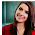This blog is under construction

## Wednesday, 2 October 2013

### Analog clock in c - Simplified

C program implement analog clock

#include <stdio.h>
#include <conio.h>
#include <string.h>
#include <math.h>
#include <time.h>
#include <graphics.h>
#include <dos.h>

/*
* calculates the position for minute and second needle.
* 360 to 90 degree is equivalent to 45 to 0 th minute
* in clock.  So, store the points in reverse direction(45 to 0)
* Once 0 is reached, we need to store points corresponds to
* 84th degree to 0 degree in reverse direction(59 to 46). So,
* totally we will get 60 points which corresponds to 60
* minute or second position in clock.
*/
void minSecCalc(int xrad, int midx, int midy, int x, int y) {
int i, j = 45;
for (i = 360; i >= 0; i = i - 6) {
x[j] = midx - (xrad * cos((i * 3.14) / 180));
y[j--] = midy - (xrad * sin((i * 3.14) / 180));
j = (j == -1) ? 59:j;
}
return;

}

/*
* find the points at 0, 30, 60,.., 360 degrees
* on the given circle.  x value correponds to
* radius * cos(angle) and y value corresponds
* to radius * sin(angle).  Numbers in the clock
* are written using the above manipulated x and
* y values.  And the hour needle movement
* is based on this
*/

void calcPoints(int radius, int midx, int midy, int x, int y) {
int x1, y1;
/* 90, 270, 0, 180 degrees */
x = midx, y = midy - radius;
x = midx, y = midy + radius;
x = midx + radius, y = midy;
x = midx - radius, y = midy;

/* 30, 150, 210, 330 degrees */
x1 = (int) ((radius / 2) * sqrt(3));
y1 = (radius / 2);
x = midx + x1, y = midy - y1;
x = midx + x1, y = midy + y1;
x = midx - x1, y = midy + y1;
x = midx - x1, y = midy - y1;

/* 60, 120, 210, 300 degrees */
x1 = radius / 2;
y1 = (int) ((radius / 2)  * sqrt(3));
x = midx + x1, y = midy - y1;
x = midx + x1, y = midy + y1;
x = midx - x1, y = midy + y1;
x = midx - x1, y = midy - y1;
return;
}

int main() {
/* request auto detection */
int gdriver = DETECT, gmode, err, tmp;
int i, j, midx, midy, radius, hr, min, sec;
int x, y, minx, miny;
int hrx, hry, secx, secy;
int secx1, secy1;
char str;
time_t t1;
struct tm *data;

/* initialize graphic mode */
initgraph(&gdriver, &gmode, "C:/TC/BGI");
err = graphresult();

if (err != grOk) {
/* error occurred */
printf("Graphics Error: %s",
grapherrormsg(err));
return 0;
}

/* mid position in x and y -axis */
midx = getmaxx() / 2;
midy = getmaxy() / 2;

radius = 200;

/* position to locate numbers in clock */
calcPoints(radius - 30, midx, midy, x, y);

/* gets position for hour needle */
calcPoints(radius - 90, midx, midy, hrx, hry);

/* gets position for minute needle */
minSecCalc(radius - 50, midx, midy, minx, miny);

/* gets position for second needle */
minSecCalc(radius - 70, midx, midy, secx, secy);

while (!kbhit()) {
setlinestyle(SOLID_LINE, 1, 3);
settextstyle(TRIPLEX_FONT, 0, 3);

/* draws frame of the clock */
circle(midx, midy, radius);

/* place the numbers  in clock */
for (j = 0; j < 12; j++) {
if (j == 0) {
sprintf(str, "%d", 12);
} else {
sprintf(str, "%d", j);
}
settextjustify(CENTER_TEXT, CENTER_TEXT);
moveto(x[j], y[j]);
outtext(str);
}

/* get the current time using time() API */
t1 = time(NULL);
data = localtime(&t1);

/* draw the second needle in clock */
sec = data->tm_sec % 60;
line(midx, midy, secx[sec], secy[sec]);

/* draw the minute needle in clock */
min = data->tm_min % 60;
line(midx, midy, minx[min], miny[min]);

/* draw the hour needle in clock */
hr = data->tm_hour % 12;
line(midx, midy, hrx[hr], hry[hr]);
delay(1000);
cleardevice();
}

getch();

/* deallocate memory allocated for graphic screen */
closegraph();
return 0;
}

Output: (analog clock in c)

#### 2 comments:

1.2.It has been simply incredibly generous with you to provide openly what exactly many individuals would’ve marketed for an eBook to end up making some cash for their end, primarily given that you could have tried it in the event you wanted.

Data Science Interview Questions

Angular JS Interview Questions

Big Data Training in Velachery

AWS Certified Developer

Best Resource of Learning Devops

Blueprism Interview Questions

Automation Anywhere Interview Questions